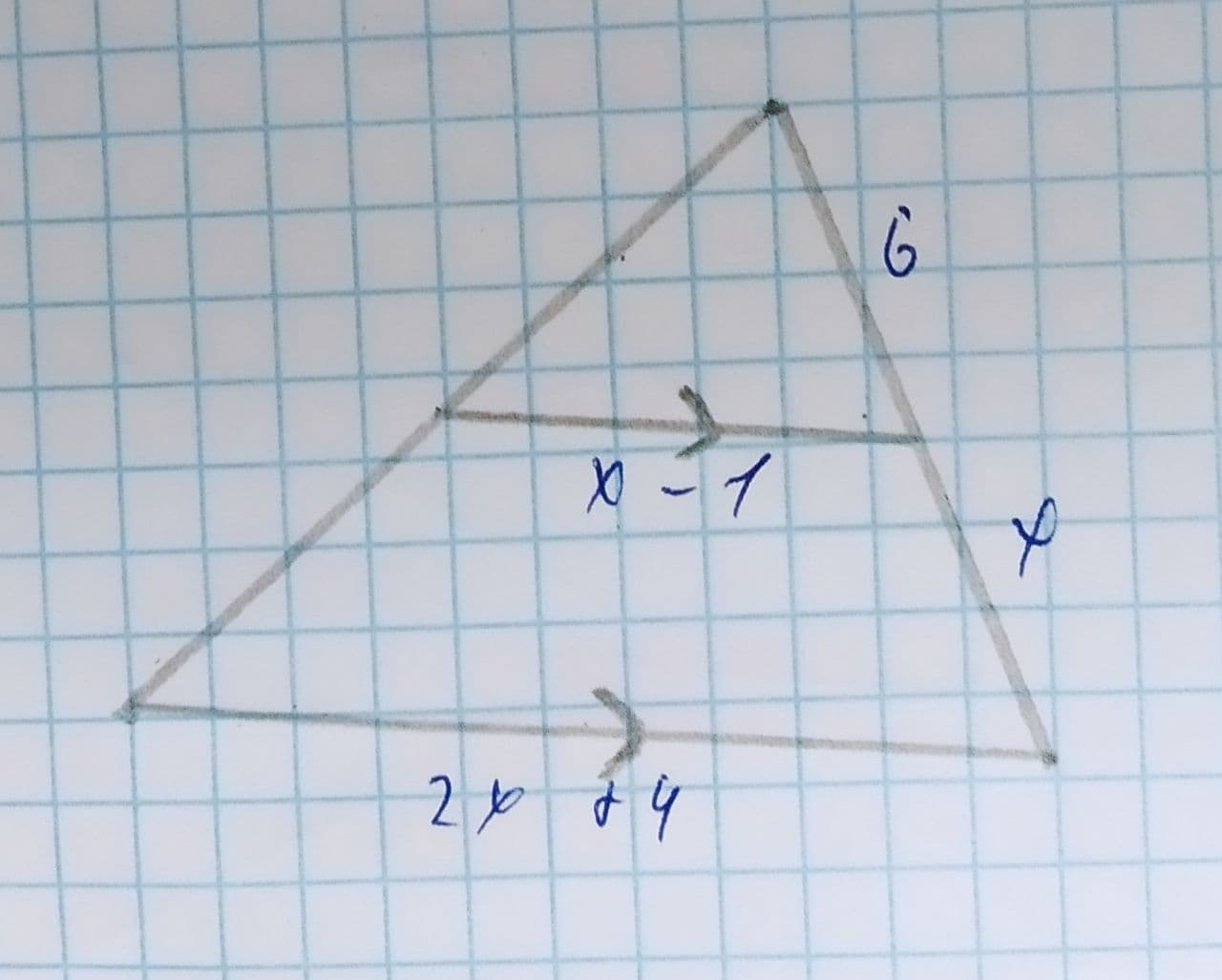Amari Flowers

2021-08-09

Use similarity in figures to solve for the value of the xMitchel Aguirre

Here $DE\parallel AC$
From the figure
$\frac{BE}{BC}=\frac{DE}{AC}$
$⇒\frac{6}{6+x}=\frac{x-1}{2x+4}$
$⇒6\left(2x+4\right)=\left(6+x\right)\left(x-1\right)$
$⇒12x+24=6x-6+{x}^{2}-x$
$⇒12x+24=5x+{x}^{2}-6$
$⇒{x}^{2}+5x-6-12x-24=0$
$⇒{x}^{2}-7x-30=0$
$⇒{x}^{2}-10x+3x-30=0$
$⇒x\left(x-10\right)+3\left(x-10\right)=0$
$⇒\left(x-10\right)\left(x+3\right)=0$
$⇒$ either x-10=0 or, x+3=0
$⇒x=10⇒x=-3$
x=-3 is not possitive since length is non negative
Hence x=10

Do you have a similar question?